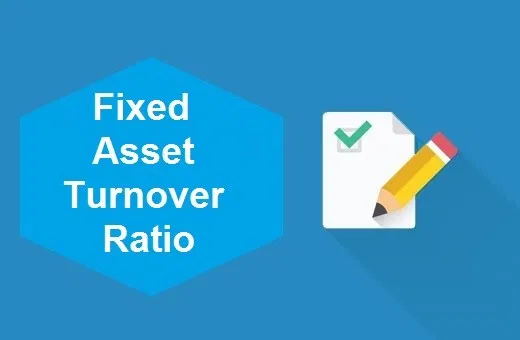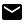# The Asset Turnover Ratio: An Important Ratio for Small Businesses

Updated July 31, 2020

Accounting ratios are an important measurement of business efficiency and profitability. A must for larger businesses, even small businesses will find accounting ratios effective.

One ratio that businesses of all sizes may find helpful is the asset turnover ratio. The asset turnover ratio measures how efficiently a business uses their assets to create sales. Learn what this ratio measures and how the information calculated can help your business.The fixed asset turnover ratio is a task that should be checked off your to-do list. Source: investopedia.com.

## Overview: What is the asset turnover ratio?

The asset turnover ratio compares sales revenue to total assets. While that’s simple enough, the results provided by the asset turnover ratio can provide an insight into your business operations that can directly affect future decision-making.

For example, as a business owner, you have assets.

For sole proprietors, those assets may consist of a checking account and a savings account, along with a computer and printer, while larger businesses may have a wide variety of assets including a building, an industrial plant, machinery and equipment, a vehicle, along with multiple bank accounts and a line of credit.

In either case, calculating the asset turnover ratio will let you know how efficiently you’re using the assets you have.

### The asset turnover ratio formula

The asset turnover formula is a simple equation you can calculate quickly. You’ll simply need the total net sales for the period in which you’re calculating the ratio and your total average assets for the period.The asset turnover ratio is a simple formula that anyone can calculate. Source: corporatefinanceinstitute.com.

Once you have these numbers, you can use the formula to calculate the asset turnover ratio for your business.

## How to calculate the asset turnover ratio

Calculating your asset turnover ratio is a quick three-step process. If you’re using accounting software, you can find these numbers on your income statement and balance sheet. If not, you’ll need to find them in your manual ledger or spreadsheet.

Even with accounting software, you’ll likely calculate the ratio separately, since very few small business accounting programs can create accounting ratios.

To get started, you follow these steps.

### Step 1. Calculate net sales

If you’re using accounting software, this is as easy as running a year-end income statement for 2019, or whatever year you’re calculating the asset turnover ratio for.

If you’re using a manual ledger system, you’ll calculate your net sales from your sales journal. Be sure your net sales total is the figure left after sales adjustments and returns have been accounted for, otherwise the ratio will be incorrect.

For the sake of completing the ratio, let’s say that your net sales for the year was \$128,000, which you’ll use when calculating the asset turnover ratio.

### Step 2. Calculate average asset value for the year

The next step will calculate your average asset value for the year. Do this by running a balance sheet dated January 1, 2019, and then running a second balance sheet dated December 31, 2019. If you’re keeping books manually, you’ll need to access both balances from your ledger.

Once you have the balances, simply add them together and divide by two to calculate your average asset value for the year. For example, if your asset total as of January 1 was \$44,000 and the ending total as of December 31 was \$51,750, you would add them together and then divide by two.

(\$44,000 + \$51,750) ÷ 2 = \$47,875

By performing this calculation, you can see that your average asset total for 2019 was \$47,875.

### Step 3. Perform the calculation

Since you have your net sales and have calculated average asset value for the year, you’re ready to calculate the asset turnover ratio.

\$128,000 ÷ \$47,875 = 2.67

Companies using their assets efficiently usually have an asset turnover ratio greater than one. An asset turnover ratio of 2.67 means that for every dollar’s worth of assets you have, you are generating \$2.67 in sales.

## Examples of the asset turnover ratio

When calculating net sales, you always need to take returns and adjustments into consideration. While accounting software will automatically calculate this for you, if you’re manually recording sales entries, you’ll need to subtract these items from gross sales to come up with an accurate net sales figure.

The same holds true when calculating asset value. When calculating total assets, include current assets such as bank accounts and accounts receivable balances, fixed assets such as equipment and machinery, along with intangible assets and investment totals.

### Asset turnover ratio: Example 1

Christine’s startup has recently taken off, with \$300,000 in gross sales. However, she has \$131,000 in returns and adjustments, making her net sales \$169,000. Her assets at the start of her business were minimal at \$40,000, but her year-end assets totaled \$127,000.

Your first calculation would be to find Christine’s average asset value for the year:

(\$40,000 + \$127,000) ÷ 2 = \$83,500

With the average total assets in place, Christine can now calculate the asset turnover ratio for her business:

\$169,000 ÷ \$83,500 = 2

Even with the high returns, Christine is earning \$2 for every dollar of assets she currently has. Since anything above one is considered good, Christine’s startup is using its assets efficiently.

### Asset turnover ratio: Example 2

Don owns a small electronics store. His sales have been steadily decreasing over the past three years. His gross sales for the year totaled \$71,000 with returns of \$11,000, making his net sales \$60,000.

Don’s assets have remained fairly steady, with a beginning asset balance of \$85,000 and an ending asset balance of \$91,000. Let’s find Don’s average asset value first:

(\$85,000 + \$91,000) ÷ \$88,000

Now we can calculate Don’s asset turnover ratio:

\$60,000 ÷ \$88,000 = 0.68

That means that for every dollar of assets Don’s business has, it’s only earning \$0.68 in sales. This result indicates that Don’s business is not using its assets efficiently.

When calculating and analyzing asset turnover ratio for your company, be sure you only compare results to those in similar industries.

For instance, a service business should only compare their ratio to similar service businesses, while hospitality and retail businesses should only compare results to other hospitality or retail companies.

## You can use the asset turnover ratio in a variety of ways

Asset utilization ratios such as the asset turnover ratio can provide a lot of information about your business. If your results are on the low side, there are ways you can increase it, such as adding a new product line or service to your business, which can help drive sales up.

You can also consider inventory and asset types you’re currently carrying on the books and see if there are ways to better utilize them, or even dispose of them.

When calculated over several years, your average asset turnover ratio can help to pinpoint business efficiency trends and spot problem areas before they become a major issue. However you use the asset turnover ratio for your business, calculating this valuable metric is important to optimize business performance.

### LOTS TO CONSIDER, LET US HELP

Get The Blueprint’s latest recommendations by signing up to our free newsletter.The Motley Fool has a Disclosure Policy. The Author and/or The Motley Fool may have an interest in companies mentioned.